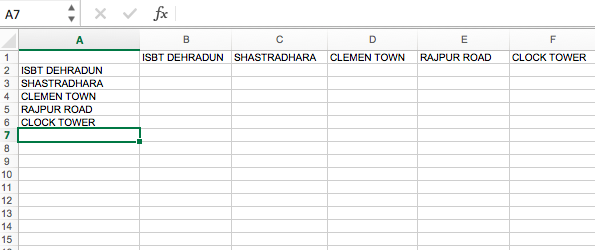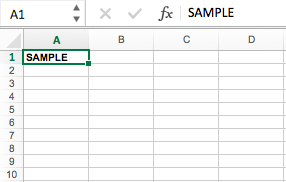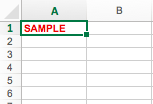# Writing to an excel sheet using Python

• Difficulty Level : Basic
• Last Updated : 29 Jul, 2019

Using `xlwt` module, one can perform multiple operations on spreadsheet. For example, writing or modifying the data can be done in Python. Also, the user might have to go through various sheets and retrieve data based on some criteria or modify some rows and columns and do a lot of work.

Let’s see how to create and write to an excel-sheet using Python.

Code #1 :

 `# Writing to an excel  ` `# sheet using Python ` `import` `xlwt ` `from` `xlwt ``import` `Workbook ` ` `  `# Workbook is created ` `wb ``=` `Workbook() ` ` `  `# add_sheet is used to create sheet. ` `sheet1 ``=` `wb.add_sheet(``'Sheet 1'``) ` ` `  `sheet1.write(``1``, ``0``, ``'ISBT DEHRADUN'``) ` `sheet1.write(``2``, ``0``, ``'SHASTRADHARA'``) ` `sheet1.write(``3``, ``0``, ``'CLEMEN TOWN'``) ` `sheet1.write(``4``, ``0``, ``'RAJPUR ROAD'``) ` `sheet1.write(``5``, ``0``, ``'CLOCK TOWER'``) ` `sheet1.write(``0``, ``1``, ``'ISBT DEHRADUN'``) ` `sheet1.write(``0``, ``2``, ``'SHASTRADHARA'``) ` `sheet1.write(``0``, ``3``, ``'CLEMEN TOWN'``) ` `sheet1.write(``0``, ``4``, ``'RAJPUR ROAD'``) ` `sheet1.write(``0``, ``5``, ``'CLOCK TOWER'``) ` ` `  `wb.save(``'xlwt example.xls'``) `

Output :Code #2 : Adding style sheet in excel

 `# importing xlwt module ` `import` `xlwt ` ` `  `workbook ``=` `xlwt.Workbook()  ` ` `  `sheet ``=` `workbook.add_sheet(``"Sheet Name"``) ` ` `  `# Specifying style ` `style ``=` `xlwt.easyxf(``'font: bold 1'``) ` ` `  `# Specifying column ` `sheet.write(``0``, ``0``, ``'SAMPLE'``, style) ` `workbook.save(``"sample.xls"``) `

Output :Code #3 : Adding multiple styles to a cell

 `# importing xlwt module ` `import` `xlwt ` ` `  `workbook ``=` `xlwt.Workbook()  ` ` `  `sheet ``=` `workbook.add_sheet(``"Sheet Name"``) ` ` `  `# Applying multiple styles ` `style ``=` `xlwt.easyxf(``'font: bold 1, color red;'``) ` ` `  `# Writing on specified sheet ` `sheet.write(``0``, ``0``, ``'SAMPLE'``, style) ` ` `  `workbook.save(``"sample.xls"``) `

Output :My Personal Notes arrow_drop_up
Recommended Articles
Page :